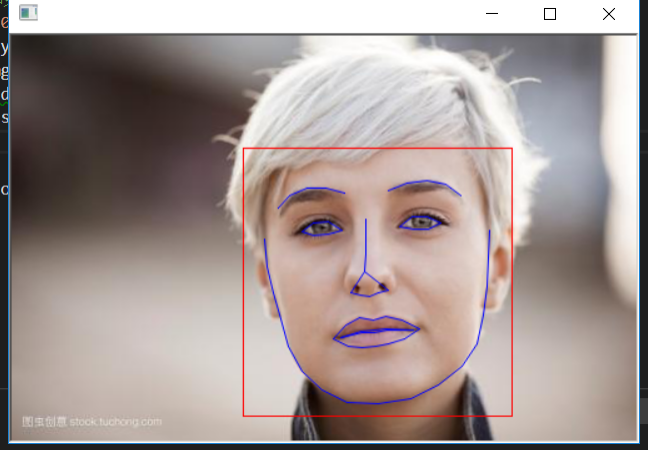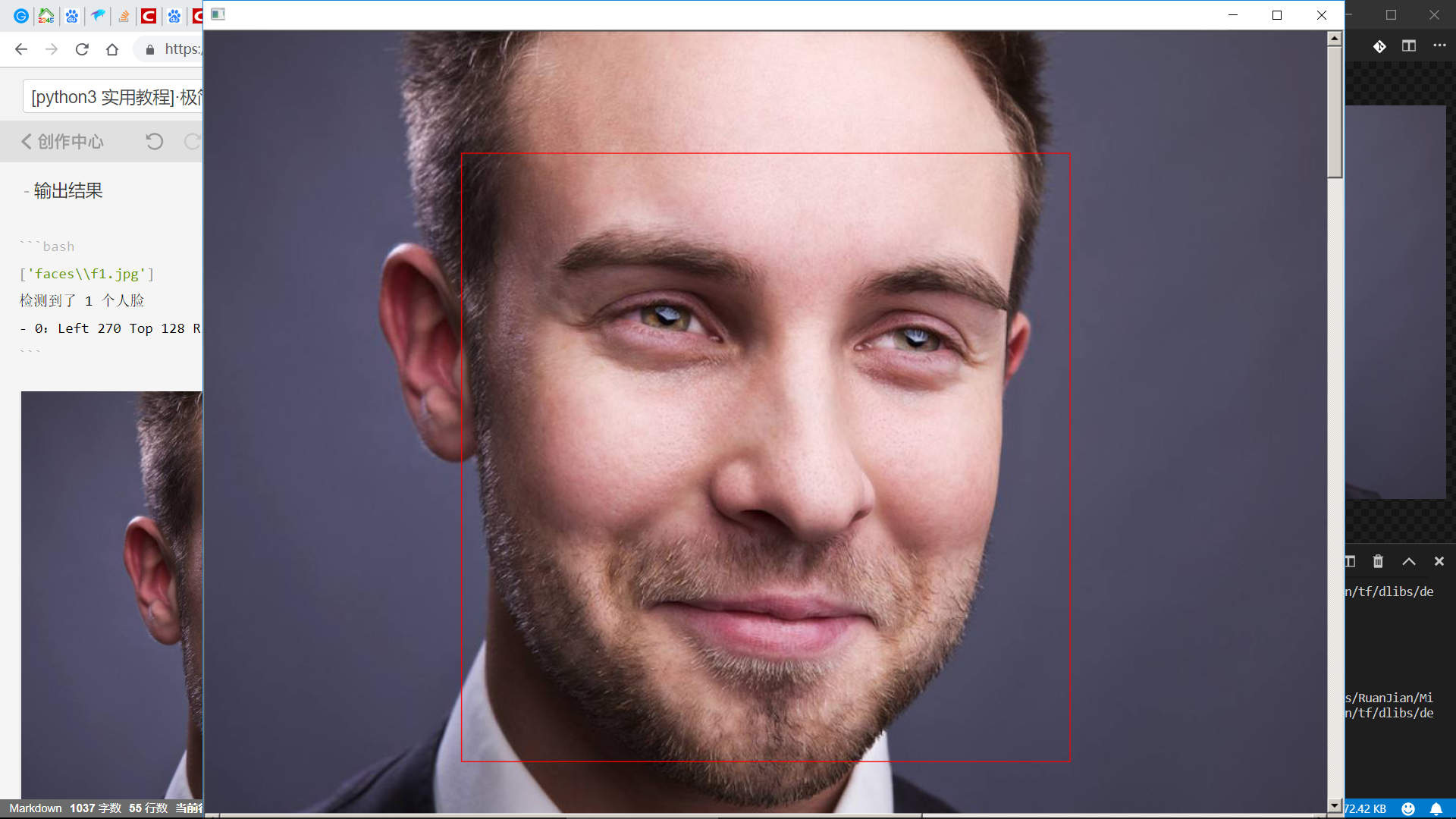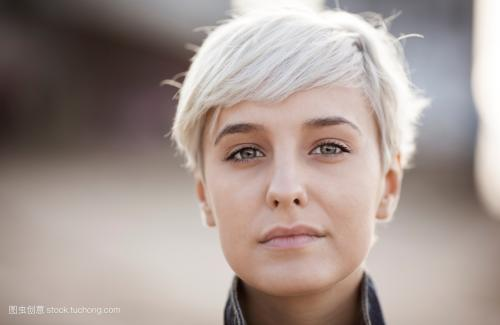# 『Python实用教程』手把手教你使用dlib实现人脸识别项目0x01：引子

0x02：环境搭建

0x03：开发实战

1.实现人脸检测标记

2.人脸特征点提取

3.人脸识别验证

0x04：后记

# 0x01：引子

Dlib是一个深度学习开源工具，基于C++开发，也支持Python开发接口，功能类似于TensorFlow与PyTorch。但是由于Dlib对于人脸特征提取支持很好，有很多训练好的人脸特征提取模型供开发者使用，所以Dlib人脸识别开发很适合做人脸项目开发。

# 0x02：环境搭建

• 安装Miniconda

• 安装dlib
注意一定要以管理员身份进入CMD，执行（如果是Linux Mac 就使用 sudo）
``````conda install -c conda-forge dlib
``````
• 需要imageio 库，可以使用下述命令安装
``````conda install imageio
``````

# 0x03：开发实战

## 1.实现人脸检测标记

face_test.py

``````import dlib
import glob

detector = dlib.get_frontal_face_detector()
win = dlib.image_window()

path = "f1.jpg"
dets = detector(img)
print('检测到了 %d 个人脸' % len(dets))
for i, d in enumerate(dets):
print('- %d：Left %d Top %d Right %d Bottom %d' % (i, d.left(), d.top(), d.right(), d.bottom()))

win.clear_overlay()
win.set_image(img)
dlib.hit_enter_to_continue()
``````## 2.人脸特征点提取

``````import dlib
import glob

detector = dlib.get_frontal_face_detector()
win = dlib.image_window()

predictor_path = 'shape_predictor_68_face_landmarks.dat'
predictor = dlib.shape_predictor(predictor_path)

path = "f2.jpg"
dets = detector(img)
print('检测到了 %d 个人脸' % len(dets))

for i, d in enumerate(dets):
print('- %d: Left %d Top %d Right %d Bottom %d' % (i, d.left(), d.top(), d.right(), d.bottom()))
shape = predictor(img, d)
# 第 0 个点和第 1 个点的坐标
print('Part 0: {}, Part 1: {}'.format(shape.part(0), shape.part(1)))
win.clear_overlay()
win.set_image(img)
dlib.hit_enter_to_continue()
``````## 3.人脸识别验证

face_recognition.py

``````import dlib
import glob
import numpy as np

detector = dlib.get_frontal_face_detector()
predictor_path = 'shape_predictor_68_face_landmarks.dat'
predictor = dlib.shape_predictor(predictor_path)
face_rec_model_path = 'dlib_face_recognition_resnet_model_v1.dat'
facerec = dlib.face_recognition_model_v1(face_rec_model_path)

def get_feature(path):
dets = detector(img)
print('检测到了 %d 个人脸' % len(dets))
# 这里假设每张图只有一个人脸
shape = predictor(img, dets)
face_vector = facerec.compute_face_descriptor(img, shape)
return(face_vector)

def distance(a,b):
a,b = np.array(a), np.array(b)
sub = np.sum((a-b)**2)

path_lists1 = ["f1.jpg","f2.jpg"]
path_lists2 = ["赵丽颖照片.jpg","赵丽颖测试.jpg"]

feature_lists1 = [get_feature(path) for path in path_lists1]
feature_lists2 = [get_feature(path) for path in path_lists2]

print("feature 1 shape",feature_lists1.shape)

out1 = distance(feature_lists1,feature_lists1)
out2 = distance(feature_lists2,feature_lists2)

print("diff distance is",out1)
print("same distance is",out2)

out1 = distance(feature_lists1,feature_lists1)
out2 = distance(feature_lists2,feature_lists2)

``````

``````检测到了 1 个人脸

feature 1 shape (128, 1)

diff distance is 0.254767715912
same distance is 0.0620976363391``````

``````def classifier(a,b,t = 0.09):
if(distance(a,b)<=t):
ret = True
else :
ret = False
return(ret)

print("f1 is 赵丽颖",classifier(feature_lists1,feature_lists2))
print("f2 is 赵丽颖",classifier(feature_lists1,feature_lists2))
print("赵丽颖照片.jpg is 赵丽颖测试.jpg",classifier(feature_lists2,feature_lists2))``````

``````f1 is 赵丽颖 False
f2 is 赵丽颖 False